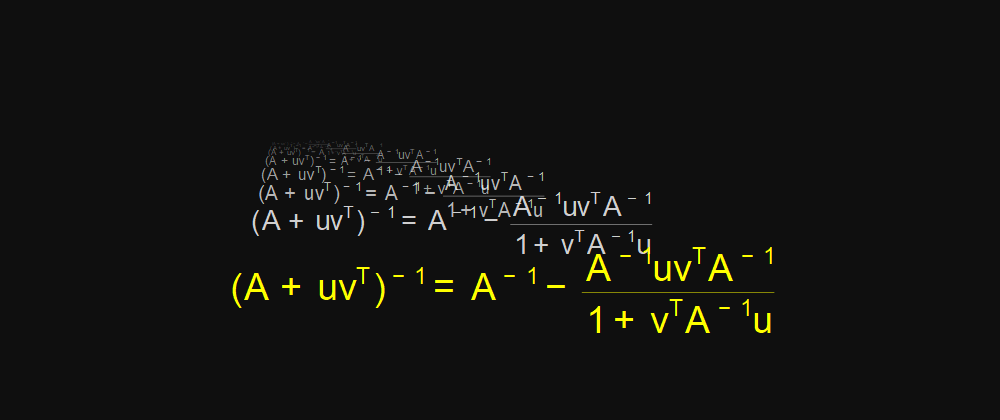## Julia Community 🟣# Exploring the Matrix Inversion Lemma in Julia

Let   $A$ be a square matrix of size  $n$  that is invertible, and let  $u$  and $v$   be non-zero column vectors of size $n$  . The matrix  $A + u v^T$  is invertible if and only if the equation  $v^TA^{−1}u \neq −1$ is true. In such a case, the inverse of   $A + u v^T$  can be determined using the following method:

$(A + uv^T)^{-1} = A^{-1} - \frac{A^{-1}uv^T A^{-1}}{1+v^TA^{−1}u}$

The above formula can be coded in Julia as

using LinearAlgebra

N = 4
A = rand(N,N)
Ainv = inv(A)

println("A = ")
display(Ainv)

u = rand(N,1)
v = rand(N,1)

B = A + u*v'

println("A + u*v' = ")
display(B)

Binv = inv(B)

if !isequal(-1,dot(v,Ainv*u))
C = Ainv - Ainv*u*v'*Ainv/(1 + dot(v,Ainv*u))
end

println("Crude Inverse")
display(Binv)

println("Inverse calculated with Matrix Inversion Lemma")
display(C)

println("Are the matrices equal")
isapprox(Binv,C)